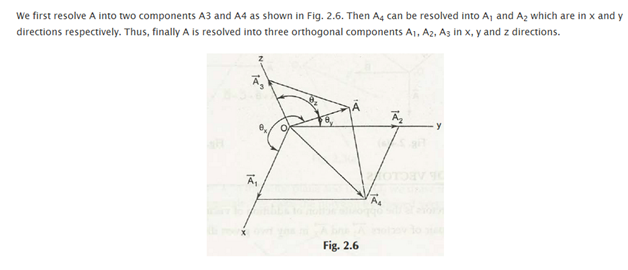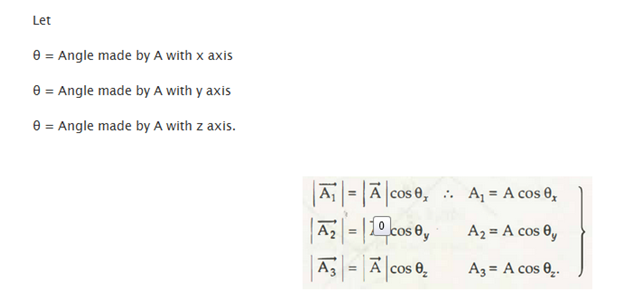Resolution of vectors can indicate lot of different things, but then again it boils down to a procedure in which one vector is broken down into two or more smaller vectors. All these include a process in which a vector a broken into two different components. Any vector directed at an angle to the horizontal can be thought of having two different components. In other words it can be said that any given vector is directed in two different dimensions which are having two components.

Components of single forces can be subdivided into parts with the help of reversing the procedure described above and then allowing them to be the outcome of two or more than two components. The technique is known as determination of the force into its constituents. It is quite helpful because it allows different force systems to be simplified into sets of forces which act in two perpendicular directions. Not only that, the system also enables the addition of forces algebraically but it can also be resolved graphically.

Uses of resolution of vectors into modules in order to determine the outcome of a set of forces –

1. Resolution of the forces into horizontal and vertical components
2. 3 concurrent forces
3. Evaluation of the outcomes by the addition of vector components### Submit Your Assignment